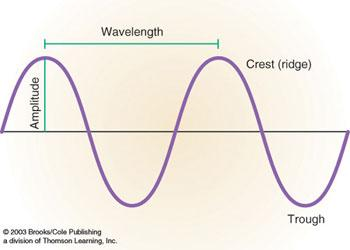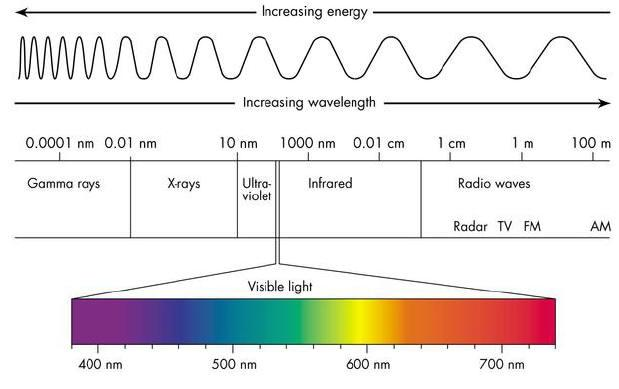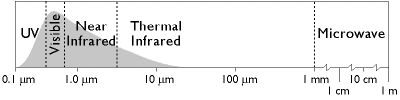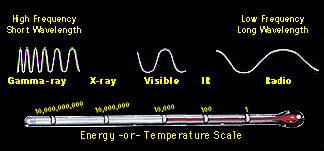# Introduction Heat energy can be transferred by 3 main physical processes: Conduction

 Remote Sensing and Electromagnetic Energy A Primer Introduction Heat energy can be transferred by 3 main physical processes: Conduction which involves contact. Heat energy is transmitted by point-to-point contact with neighboring molecules. This is exhibited in solid substances, by holding a metal rod in a fire and the heat is conducted from one end to the other. Solids are good conductors while fluids and gases (e.g. the atmosphere) are poor conductors. Convection which involves movement. Heat is transmitted by molecules moving from one place to another. This occurs in fluids and gases (e.g. atmosphere) but not solids. Examples include the daytime sea breeze and nighttime land breeze which both involve the movement of air. Radiation: transfer of heat energy without involvement of a physical substance (e.g. solid, fluid or gas) in the transmission. Thus the energy can be transmitted through a vacuum (space) or a transparent medium (atmosphere). The incoming solar energy is an example of radiation. Electromagnetic energy - Basics Remote sensors aboard satellites are designed to observe and record energy reflected (i.e. visible satellite imagery) and energy emitted (i.e. Infrared or IR satellite imagery) by or from the earth/atmosphere system. In the simplest terms, radiation is a form of energy. This energy is emitted by all substances above absolute zero (0 K, -273.15°C or -459.7°F). This energy is transmitted in the form of a wave. The specific characteristics of the wave vary, but follow the form in figure 1 below: wavelength, l(meters) amplitude, a (meters) velocity, v (m/s) waves move at the speed of light (c ~ 3.00 × 108 m/s) frequency, f where v = (f) * (l) Figure – 1 Characteristics of Electromagnetic (EM) Energy These waves of energy are constantly moving all around the universe in the form of radio and TV transmissions, gamma and X-rays from space, and heat in the earth system. The energy we will be discussing ultimately is generated by the sun. These energy waves consist of both electric and magnetic wave characteristics moving perpendicular to each other. These waves are classified by their frequency (i.e. ranging from high to low) or their wavelength (i.e. ranging from short to long). However, all EM energy waves travel at the speed of light, regardless of their frequency or wavelength. The entire electromagnetic (EM) spectrum contains all the differing wavelengths, and this is depicted in figure 2. The shorter wavelengths (e.g. gamma or x-rays) have more energy, while the longer wavelengths (i.e. lower frequency) have less energy (e.g. TV and radio waves). The visible wavelengths lie somewhat in the middle, as depicted figure 2:Fig 2 - Electromagnetic spectrum of the sun. http://www.antonine-education.co.uk/physics_gcse/Unit_1/Topic_5/em_spectrum.jpg To summarize, the EM spectrum can be expressed in terms of energy, wave-length or frequency. Mathematically, this is stated as: v = (f) * (l) Each parameter used to view or express the EM spectrum are inter-related as noted in the equation above. Figure 3 clearly relates energy and wavelength, with the short wave length being the most energetic.Fig – 3 EM spectrum relationships Courtesy:http://imagine.gsfc.nasa.gov/index.html Download 95.37 Kb.Share with your friends: